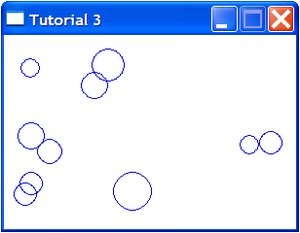# Randomness

2 Feb 2010

Most simulations will start with a random (or semi-random) initial configuration. Some will introduce random noise to mimic real life uncertainty. In this tutorial, we will:

• Use Python’s random module
• Create multiple Particle objects

While this tutorial isn't specific to Pygame, it is import for our simulation. If you're already familiar with Python's random module then you can skip this tutorial. As before, the final code can be found by clicking the Code on Github link above.

## The random module

To generate random numbers in Python (not just Pygame), we need to import the random module. This module is part of the main Python program so you don't need to download anything extra.

``import random``

We now have access to various functions that generate random numbers. The functions I use most often are:

• random() - returns a random number between 0.0 and 1.0
• uniform(a, b) - returns a random number between a and b
• randint(a, b) - returns a random integer between a and b
• choice(list) - returns a random element from the list
• shuffle(list) - rearranges the order of the list

For the full range of functions see the Python documentation. For now we just need random.randint().

The code below demonstrates how to create a circle with a radius between 10 and 20 pixels (inclusive) and with a random position on the screen. To ensure that the no part of the circle is off-screen rather than picking a value between 0 and the window's dimensions, we reduce the range of values by the size of circle.

``````size = random.randint(10, 20)
x = random.randint(size, width - size)
y = random.randint(size, height - size)
my_random_particle = Particle((x, y), size)
my_random_particle.display()``````

Now, every time the program is run, unless we are very lucky, it will display a circle with a different size and position.

## Creating multiple particles

Since we're using variables instead of constants for the particle's position, we can easily add multiple particles to the screen. This will be useful later because most simulations of particles are likely to have more than one. We deal with multiple Particle objects by creating an array and filling it with random Particles using a for loop. Replace the above code with the following:

``````number_of_particles = 10
my_particles = []

for n in range(number_of_particles):
size = random.randint(10, 20)
x = random.randint(size, width-size)
y = random.randint(size, height-size)
my_particles.append(Particle((x, y), size))``````

To display all the particles we need to change the line:

``my_random_particle.display()``

To:

``````for particle in my_particles:
particle.display()``````

For the full program (rearranged to be slightly more logical) see the link to Github at the top of this post. If you run the program, you will see ten randomly placed and randomly sized circles, some of which may overlap. You might want to avoid having the circles overlap, but I’ll leave you to work out how to do that.In the next tutorial, the simulation will become a bit more interesting as things get dynamic and the particles move.

#### Sabun on 2 Aug 2012, 5:34 a.m.

Awesome! It's so easy to do with python/pygame that I'm surprised. I want to thank you for taking the time to make this tutorial. I'm learning a lot of new things from this. Works great with IDLE 2.7 on Ubuntu 12.04 if anyone is wondering :)

#### Anonymous on 17 Oct 2013, 2:55 p.m.

hi

i am stuck

for n in range(number_of_particles):
size = random.randint(10, 20)
x = random.randint(size, width-size)
y = random.randint(size, height-size) < get erro, dose not match outer inden level
my_particles.append(Particle((x, y), size))
using IDLE 3 on respberry pi b
any help would be much appreciated
p.s have checked ana recheced for typ'o

#### Peter on 17 Oct 2013, 6:07 p.m.

It looks like you have an issue with whitespace. Make sure you are using all spaces or all tabs and have the same number of spaces or tabs in each line.

#### Anonymous on 10 Nov 2013, 3:46 a.m.

First of all, I would like to thank you for posting such a great an comprehensive tutorial, but it seems to have some problems in python 3.3. I have successfully debugged a good deal of the script, but it is rather challenging when dealing with things I have never used before. Could you please update or add footers with updated script? Thanks.

#### Anonymous on 20 May 2014, 6:03 a.m.

Things going great..however, when i try to print the my_particles
list, it gets me some wierd code..how am i supposed to fix that..??

#### Iavor on 3 Apr 2015, 4:23 a.m.

What sort of weird code are you getting?

#### Carlos on 21 Oct 2016, 10:51 p.m.

Great tutorials, it gets so easy and fun to learn Python with this awesome tutorials :)

Thanks a lot.

#### Marxist-Leninist on 5 Nov 2017, 5:49 a.m.

For any person stuggling with making the circles genrate without overlapping, here is the soultion I've came up with:

for n in range(number_of_particles): # for loop starts
size = random.randint(10, 20)
x = random.randint(size, width-size) #randomize x position
y = random.randint(size, height-size) #randomize y position

for i in range(len(my_particles)):
while my_particles[i].x - size <= x <= my_particles[i].x + size:
x = random.randint(size, width-size)
while my_particles[i].y - size <= y <= my_particles[i].y + size:
y = random.randint(size, height - size)

my_particles.append(Particle((x,y), size))

#### Tom on 23 Mar 2019, 12:23 p.m.

for particle in my_particles:

Invalid syntax pointing to “:” ...I can’t see the issue here?